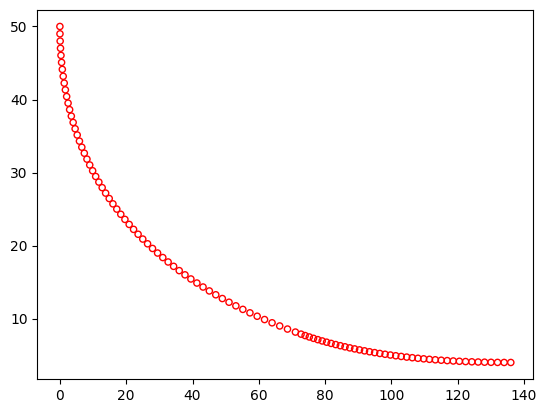# BNH¶

Binh and Korn defined the following test problem in  with 2 objectives and 2 constraints:

Definition

\begin{equation} \newcommand{\boldx}{\mathbf{x}} \begin{array} \mbox{Minimize} & f_1(\boldx) = 4x_1^2 + 4x_2^2, \\ \mbox{Minimize} & f_2(\boldx) = (x_1-5)^2 + (x_2-5)^2, \\ \mbox{subject to} & C_1(\boldx) \equiv (x_1-5)^2 + x_2^2 \leq 25, \\ & C_2(\boldx) \equiv (x_1-8)^2 + (x_2+3)^2 \geq 7.7, \\ & 0 \leq x_1 \leq 5, \\ & 0 \leq x_2 \leq 3. \end{array} \end{equation}

Optimum

The Pareto-optimal solutions are constituted by solutions $$x_1^{\ast}=x_2^{\ast} \in [0,3]$$ and $$x_1^{\ast} \in [3,5]$$, $$x_2^{\ast}=3$$. These solutions are marked by using bold continuous curves. The addition of both constraints in the problem does not make any solution in the unconstrained Pareto-optimal front infeasible. Thus, constraints may not introduce any additional difficulty in solving this problem.

Plot

:

from pymoo.factory import get_problem
from pymoo.util.plotting import plot

problem = get_problem("bnh")
plot(problem.pareto_front(), no_fill=True)[ ]: